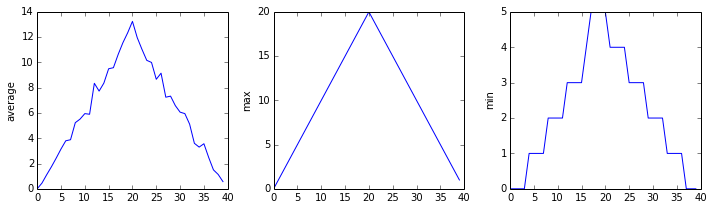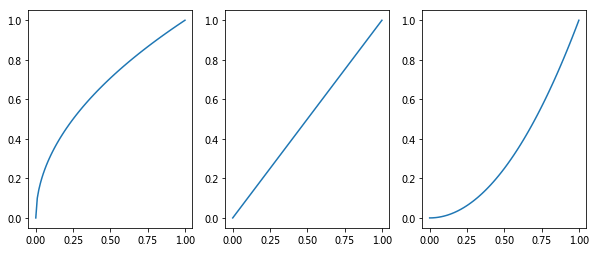#### python中怎么用matplotlib在一行里生成多个图？

统计/机器学习 数据可视化 Python    浏览次数：4417        分享

0yangyang   2018-08-15 21:55

##### 1个回答
4

import matplotlib.pyplot as plt
import numpy as np

x = np.linspace(0, 1, 100)
plt.figure(figsize=(10, 4))
# 一共有一行三列，现在画第一幅图
plt.subplot(1, 3, 1)
plt.plot(x, x ** 0.5)
# 一共有一行三列，现在画第二幅图
plt.subplot(1, 3, 2)
plt.plot(x, x ** 1)
# 一共有一行三列，现在画第三幅图
plt.subplot(1, 3, 3)
plt.plot(x, x ** 2)
plt.show()SofaSofa数据科学社区DS面试题库 DS面经abuu   2018-08-20 09:43相关主题